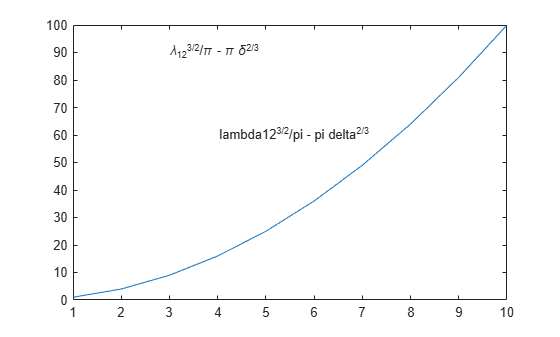# texlabel

Format text with TeX characters

## Syntax

``txt = texlabel(f)``
``txt = texlabel(f,'literal')``

## Description

example

````txt = texlabel(f)` converts the MATLAB® expression `f` into the TeX equivalent for use in text. `texlabel` converts Greek variable names (for example, lambda, delta, and so on) into a character vector that is displayed as Greek letters. The `txt` output is useful as an argument to annotation functions such as `title`, `xlabel`, and `text`.```

example

````txt = texlabel(f,'literal')` interprets Greek variable names literally.```

## Examples

collapse all

Plot a line. Create text that contains TeX characters and add the text to the line plot.

```plot((1:10).^2) txt = texlabel('lambda12^(3/2)/pi - pi*delta^(2/3)'); text(3,90,txt)```If you include the `'literal'` argument, `texlabel` interprets Greek variable names literally. Add text with the literal variable names to the line plot.

`text(4,60,texlabel('lambda12^(3/2)/pi - pi*delta^(2/3)','literal'))`## Input Arguments

collapse all

Input MATLAB expression, specified as a character vector or string scalar.

Example: `'theta (degrees)'`